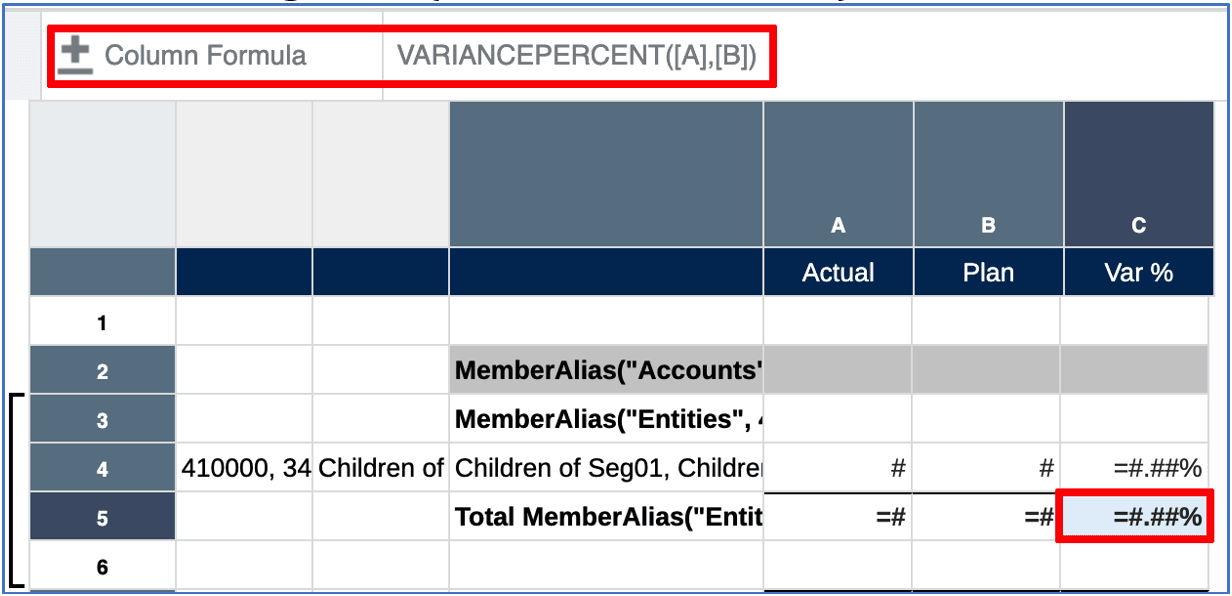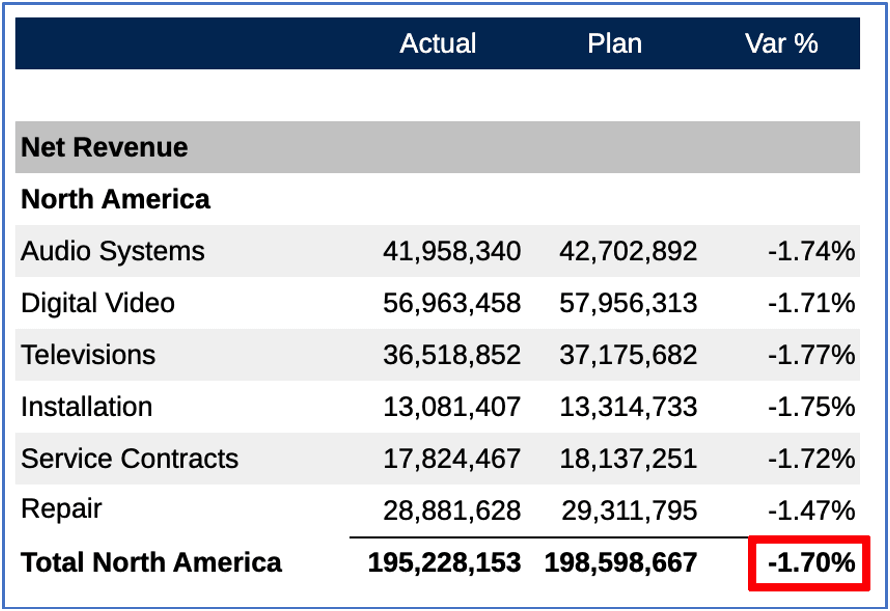## Grouping and Grid Formulas

In a grid with formulas on both the rows and columns, where grouping is applied to one of the axes, and intersecting opposite axis formula will still calculate independently of the grouping.

For example, with a row formula to sum Segments (within each Entity), where grouping is applied, and a column formula to calculate the variance % based on two columns:

• Row 5 has a SUM formula, which sums row 4 and is included in a grouping. The formula will return subtotals for the Entity groupings.

• Columns C has a Variance% formula, which calculates variance between columns A and B. Note in the formula bar that for the intersecting cell C1, the columns formula is being used (vs. the row formula).Note in a portion of the resulting grid preview, that while the subtotal for the Entity "North America" is summed for the "Actual" and "Plan" columns, the "Var%" column is calculated based on the variance of the subtotal row and is not summed from the individual Segment variances.